## 6.10The nature of viscosity

Before discussing turbulence further, it is useful to examine the origins of viscosity. Viscosity is introduced in Sec. 2.12 of this book as part of the Newtonian constitutive model. The model was originally phenomenological, but was later derived directly from kinetic theory which describes a gas as a number of submicroscopic particles, e.g. atoms or molecules, in random motion.

The kinetic view of viscosity imagines a ﬂuid in two dimensions,and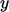, subjected to a shear force in the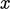-direction. Although the mean ﬂow is in the-direction, particles move in the-direction due to random ﬂuctuations with a mean speed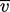.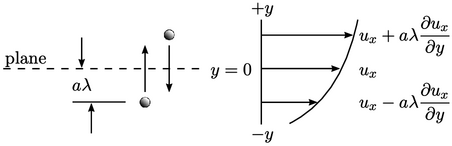Consider a plane at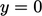. A particle will pass through the plane if its path towards it is not interrupted by a collision which sends it moving away from the plane. Particles passing through the plane arrive from an average distance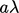, where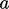is some factor of the average distance travelled by a moving particle between successive collisions, the mean free path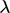.

From kinetic theory, the mass ﬂow rate of particles passing through a surface of unit area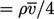. The mean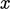-velocity of particles crossing the plane from the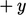-direction is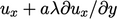; similarly from the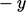-direction, it is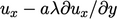.

The net-momentum of the particles, positive on the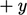side of the plane is then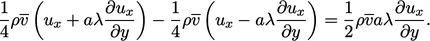(6.16)

The net momentum is equivalent to the shear stress on the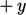side of the plane,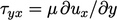, as described in Sec. 2.6 . By comparison with Eq. (6.16 ), the dynamic viscosity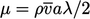in terms of molecular properties. The kinematic viscosity is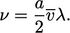(6.17)
The original analysis of Maxwell12 uses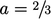in Eq. (6.17 ). It was later recognised the average distance described by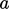was larger due to persistence of velocities, i.e. a particle will sometimes maintain a path towards the plane after a collision.

A more thorough analysis13 begins with the Boltzmann equation and applies the Chapman–Enskog expansion to ﬁrst order in Knudsen number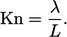(6.18)
The analysis derives the conservation laws with Newtonian and Fourier constitutive models, i.e. Eq. (2.19 ), Eq. (2.51 ), Eq. (2.41 ) and Eq. (2.54 ). Treating particles as rigid spheres and collisions as elastic, yields a value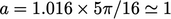, leaving a simple expression for viscosity which is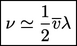(6.19)

12James Clerk Maxwell, Illustrations of the dynamical theory of gases. Part I. On the motions and collisions of perfectly elastic spheres, 1860.
13see Sydney Chapman and Thomas Cowling, The mathematical theory of non-uniform gases (3rd ed.), 1970.

Notes on CFD: General Principles - 6.10 The nature of viscosity